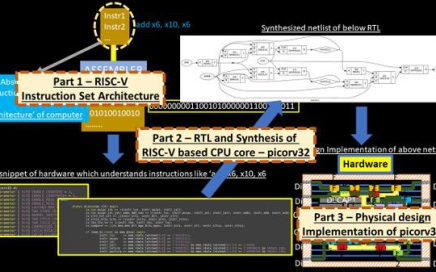From concept to algorithm – RISC-V floating point addition

First thing which comes in the title is “concept”, which is the simple one. It says, if I want to add 2 floating-point numbers, I simply add them. Second, “algorithm” is the details for a computer, how it will add 2 floating-point numbers.Third, which is “RISC-V”, which deals with binary numbers, describes how can you use same concept and algorithm to do a “binary floating-point addition”